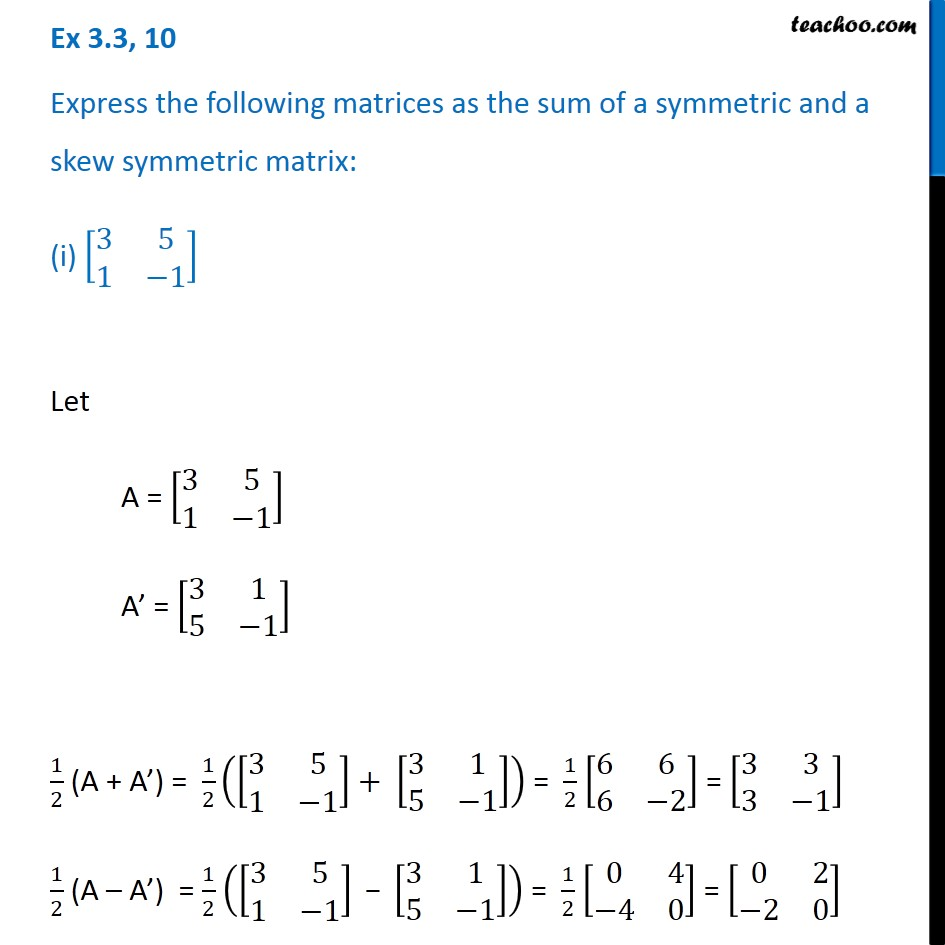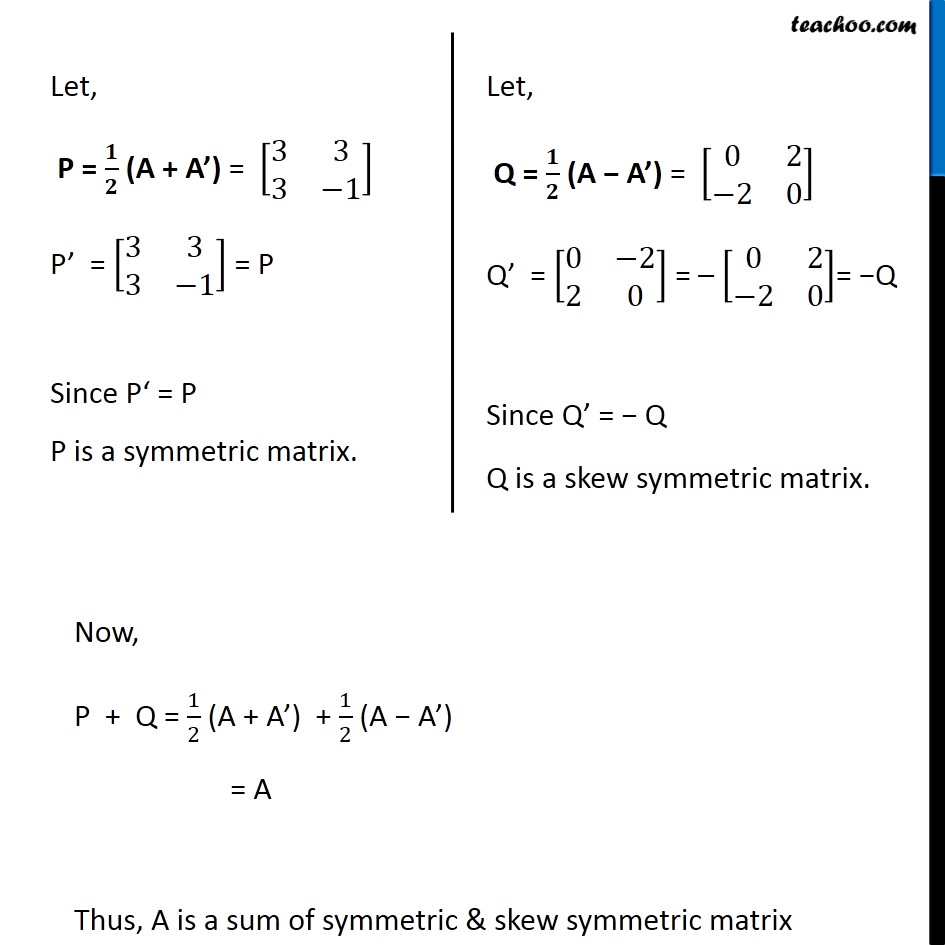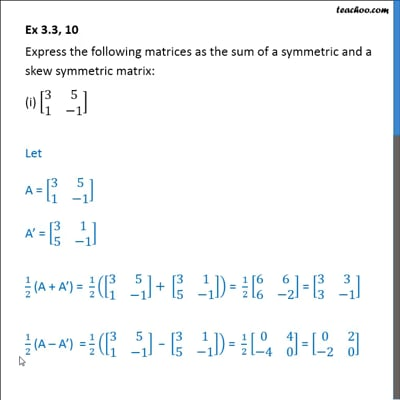Symmetric and skew symmetric matrices

Chapter 3 Class 12 Matrices
Concept wiseThis video is only available for Teachoo black users

Introducing your new favourite teacher - Teachoo Black, at only ₹83 per month

### Transcript

Ex 3.3, 10 Express the following matrices as the sum of a symmetric and a skew symmetric matrix: (i) [■8(3&5@1&−1)] Let A = [■8(3&5@1&−1)] A’ = [■8(3&1@5&−1)] 1/2 (A + A’) = 1/2 ([■8(3&5@1&−1)]+ [■8(3&1@5&−1)]) = 1/2 [■8(6&6@6&−2)] = [■8(3&3@3&−1)] 1/2 (A – A’) = 1/2 ([■8(3&5@1&−1)]" − " [■8(3&1@5&−1)]) = 1/2 [■8(0&4@−4&0)] = [■8(0&2@−2&0)] Let, P = 𝟏/𝟐 (A + A’) = [■8(3&3@3&−1)] P’ = [■8(3&3@3&−1)] = P Since P‘ = P P is a symmetric matrix. Let, Q = 𝟏/𝟐 (A − A’) = [■8(0&2@−2&0)] Q’ = [■8(0&−2@2&0)] = – [■8(0&2@−2&0)]= −Q Since Q’ = − Q Q is a skew symmetric matrix. Now, P + Q = 1/2 (A + A’) + 1/2 (A − A’) = A Thus, A is a sum of symmetric & skew symmetric matrix